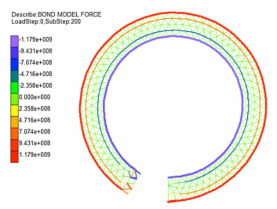News Detail

# DLSM solves large deformation problems

As a highly non-linear material with complex mechanical properties, rock and soil mass will undergo corresponding displacement deformation or damage when the force on a certain surface exceeds strength under the action of large stress, then enters the stage of large deformation. However, due to the complex process of large deformation, the current numerical simulation is still not perfect, and the existing methods cannot fully reflect its mechanical characteristics. The classical LSM can solve problems only at a fixed Poisson’s value, these models have still considered only the normal spring and central interactions between particles, but don’t overcome the limitation of Poisson's ratio.

In DLSM, the restriction on the Poisson’s ratio in classical LSM has been solved through a technique to evaluate spring deformation using the local strain technique rather than the particle displacements directly. In the developed DLSM, first, the formulation of a spring bond deformation under a large deformation is derived using polar decomposition. Next, the tangent stiffness matrix of the DLSM under a large deformation is proven equal to the stiffness matrix of the DLSM under a small deformation. Formulations to calculate the internal spring bond force and unbalanced force are also derived to solve the large deformation problems.

With the example of the cantilever beam subjected to an end moment, deformed configuration of the beam moment bending problem obtained by DLSM with the Euler method, the modified Euler method and the Newton method, and the curve of the ratio of unbalanced force was analyzed. The results show that the DLSM can solve the large deformation problems. Among them, the Euler method is simple to implement but computationally inefficient. The Newton method is the most precise, while the modified Euler method is the most robust. A combination of the modified Euler and Newton methods can result in better performance.Figure 1. Based on the Euler method to solve the characteristic result of DLSM simulation beam under the action of bending moment-bending problem.Figure 2. Based on the modified Euler method to solve the characteristic result of DLSM simulation beam under the action of bending moment-bending problem.Figure 3. Based on the Newton method to solve the characteristic result of DLSM simulation beam under the action of bending moment-bending problem

Article classification: 英文版数值验证
Share to：
Building 43, Beiyangyuan Campus, Tianjin University, Jinnan District, Tianjin
DiceTju@qq.com